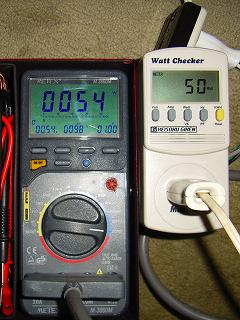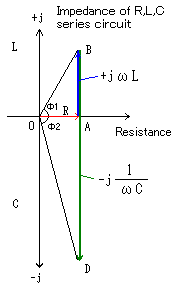# Measurement of Watt Checker Performance (2000MS1)

The eco-watt is a wattmeter on the market easily though the wattmeter is usually used for the measurement of power consumption. If the electric power of the product with small power factor is measured, the eco-watt grows the error margin and doesn't become something useful. Then, because watt checker (2000MS1) of the instrumentation technology laboratory that was able to measure power factor had been obtained, the performance was easily measured. The result was able to measure accurately even if power factor was bad (Even if it was small), and was a performance more than the price.

## Outline of watt checker (2000MS1)This photograph is the one that M-3860M of left METEX was made a wattmeter, and the one when power consumption etc. are measured by using watt checker (2000MS1) at the same time.

There is an insertion plug part on the other side of the watt checkered main body, and is an outlet of the equipment measured in the upper part of the main body. The button can be measured at once in ..pushing.. [dakede] by inserting it in these outlets.

The measurement items are eight items (voltage (V), current (A), active power (W), apparent power (VA), frequency (Hz), power factor (PF), integral power consumption (kWH), and adjusting time (H)).

There seems to be the one of the product number named TAP-TST5 though the product obtained this time was 2000MS1, too. I think that contents are maybe the same. The basic performance is the same recently though there is the one that multiplication charge named TAP-TST7 and CO2 conversion (kg) can be displayed, too.

### Time base range and measurement precision of watt checker (2000MS1)

Measurement
item
Time base range Measurement
precision(Typ.)
Measurement
precision(Max.)
Power-supply
voltage(AC,RMS)
85.0-125.0V 0.2% 1%
Power supply
current(AC,RMS)
0.00-15.00A 0.3% 1%
Active power 0-1875W 0.5% 2%
Apparent power 0-1875VA 0.5% 2%
Frequency(Hz) 47.0-63.0 0.1Hz 2%
power factor 0.00-1.00 0.01 0.03
Integral power
consumption
0.00-9999kWH 0.5% 2%
Measuring period 1Sec. -

## Result of a measurement such as various electric powers(comparison of M-3860M of watt checker and METEX)

The power factor of National fan (F-A302C) experimented on the measured equipment to the septic tank air pump of the load of the one and L character of almost one (National CL2329A) and this septic tank air pump as follows by the one of the load of C character that connected the capacitor of 18μF with the series and the one that the capacitor of 10μF200VAC of C character was completely assumed to be a load.

The value as which both of the result of a measurement were almost the same was indicated. The current became 0A why and M-3860M of METEX was not able to be measured for the load only of the capacitor.

Measurement
item
Fan
power factor
Air pump
Air pump of
C assistant
10μF capacitor
(only capacity)
Power-supply
voltage(RMS)
103V(100V) 104V(101V) 104V(101V) 103V(100V)
Power supply
current(RMS)
0.50A(0.54A) 1.26A(1.24A) 0.86A(0.99A) 0.37A(0A)
Active power 51W(54W) 48W(51W) 23W(24W) 0W(0W)
Apparent power 52VA 131VA 90VA 39W
Power
factor(PF)
0.99(1.00) 0.36(0.41) 0.25(0.25) 0.01(0.00)

Measurements without parentheses are the one of watt checker (2000MS1). Measurements in parentheses are the one of M-3860M of METEX.

Any M-3860M of METEX can be measured by voltage (V), current (A), power factor (PF), and active power's (W) being displayed at the same time even if it doesn't do.

### Theory that makes air pump used because of this experiment capacitive from inductivityIt easily explains R, L that adds the capacitor to the series, and the concept of the impedance of C series circuit in the septic tank air pump (National CL2329A).

An electric circuit of the air pump of diaphragm type's septic tank can be considered that resistance that the inductance of the coil is the main and is small is in the series. To connect the capacitor of small capacity (large reactance) with the series and to decrease power consumption to this, it planned.

Resistance R resistance is assumed to be red vector OA in a left complex impedance chart. The reactance of the coil corresponds to vector AB in blue + jωL. Therefore, the resistance of the exchange of this air pump (impedance) corresponds to the length of vector OB. (power factor is cosΦ1. )

The reactance of the connected capacitor is j(1/ωL) in the series in this air pump - of green It corresponds to the length of vector BD and it has a negative value. When you connect this with the series It becomes the vector of O-A-B-D, it becomes impedance that corresponds to the length of vector OD in the synthesis, and it becomes a capacitive load. (The power factor of the synthesis is cosΦ2.)

It is time when it is necessary to take care is near the absolute value of the reactance of the coil the reactance of the capacitor connected with the series. It is a thing that reactance is counterbalanced at this time, the resonance happens, it becomes only resistance's worth, and an excessive current flows.

Here,(ω=2πf   f is frequency.)

## Summary and conclusion

This watt checker is a standard price of about 10,000 yen, was sold at 5000 yen. I think for the price very good product performance. Eco watts compared to the difference in performance is obvious.

I think it is the best for the check on the power consumption of home appliance, the measurement of the multiplication electric power, and the measurement of the standby power requirement. However, I do not think that I project on the back side of the main body the plug, and use it easily a little. The plug can be stored or the plug with a short code is smarter.